# Electric Circuits - Online Test

Q1.
The impedance looking into nodes 1 and 2 in the given circuit is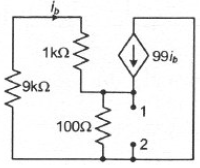Answer : Option A
Explaination / Solution: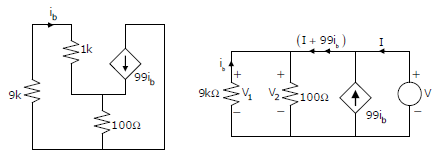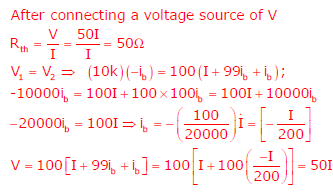Q2. In the following figure, C1 and C2 are ideal capacitors. C1 has been charged to 12 V before the ideal switch S is closed at t = 0. The current i(t) for all t is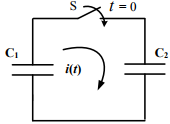Answer : Option D
Explaination / Solution:

Time constant = RC In the given circuit, R = 0 Rise time = 0; hence capacitor charges instantaneously and the current can be represented as impulse function

Q3. The average power delivered to an impedance (4 - j3)Ω by a current 5cos(100πt +100) A is
Answer : Option B
Explaination / Solution:Q4.
With 10 V dc connected at port A in the linear nonreciprocal two-port network shown below, the following were observed:
(i) 1Ω connected at port B draws a current of 3 A
(ii) 2.5Ω W connected at port B draws a current of 2 A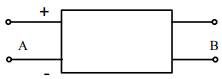For the same network, with 6 V dc connected at port A, 1Ω connected at port B draws 7/3 A. If 8 V dc is connected to port A, the open circuit voltage at port B is
Answer : Option B
Explaination / Solution:
No Explaination.

Q5.
With 10 V dc connected at port A in the linear nonreciprocal two-port network shown below, the following were observed:
(i) 1Ω connected at port B draws a current of 3 A
(ii) 2.5Ω W connected at port B draws a current of 2 AWith 10 V dc connected at port A, the current drawn by 7 Ω connected at port B is
Answer : Option C
Explaination / Solution:
No Explaination.

Q6. In the circuit shown, the three voltmeter readings are V1 = 220 V , V2 = 122 V, V3 = 136 V .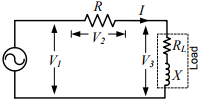If RL = 5Ω , the approximate power consumption in the load is

Answer : Option B
Explaination / Solution: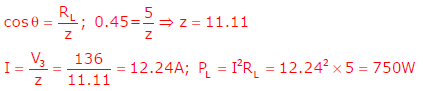Q7.
Consider a delta connection of resistors and its equivalent star connection as shown below. If all elements of the delta connection are scaled by a factor k, k>0, the elements of the corresponding star equivalent will be scaled by a factor of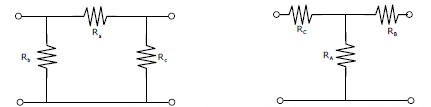Answer : Option B
Explaination / Solution:
No Explaination.

Q8. A bulb in a staircase has two switches, one switch being at the ground floor and the other one at the first floor. The bulb can be turned ON and also can be turned OFF by any one of the switches irrespective of the state of the other switch. The logic of switching of the bulb resembles
Answer : Option C
Explaination / Solution:
No Explaination.

Q9. Thyristor T in the figure below is initially off and is triggered with a single pulse of width 10µs . It is given that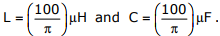Assuming latching and holding currents of the thyristor are both zero and the initial charge on C is zero, T conducts for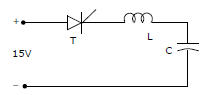Answer : Option C
Explaination / Solution:
No Explaination.

Q10. Two magnetically uncoupled inductive coils have Q factors q1 and q2 at the chosen operating frequency. Their respective resistances are R1 and R2 . When connected in series, their effective Q factor at the same operating frequency is
Answer : Option C
Explaination / Solution:
No Explaination.